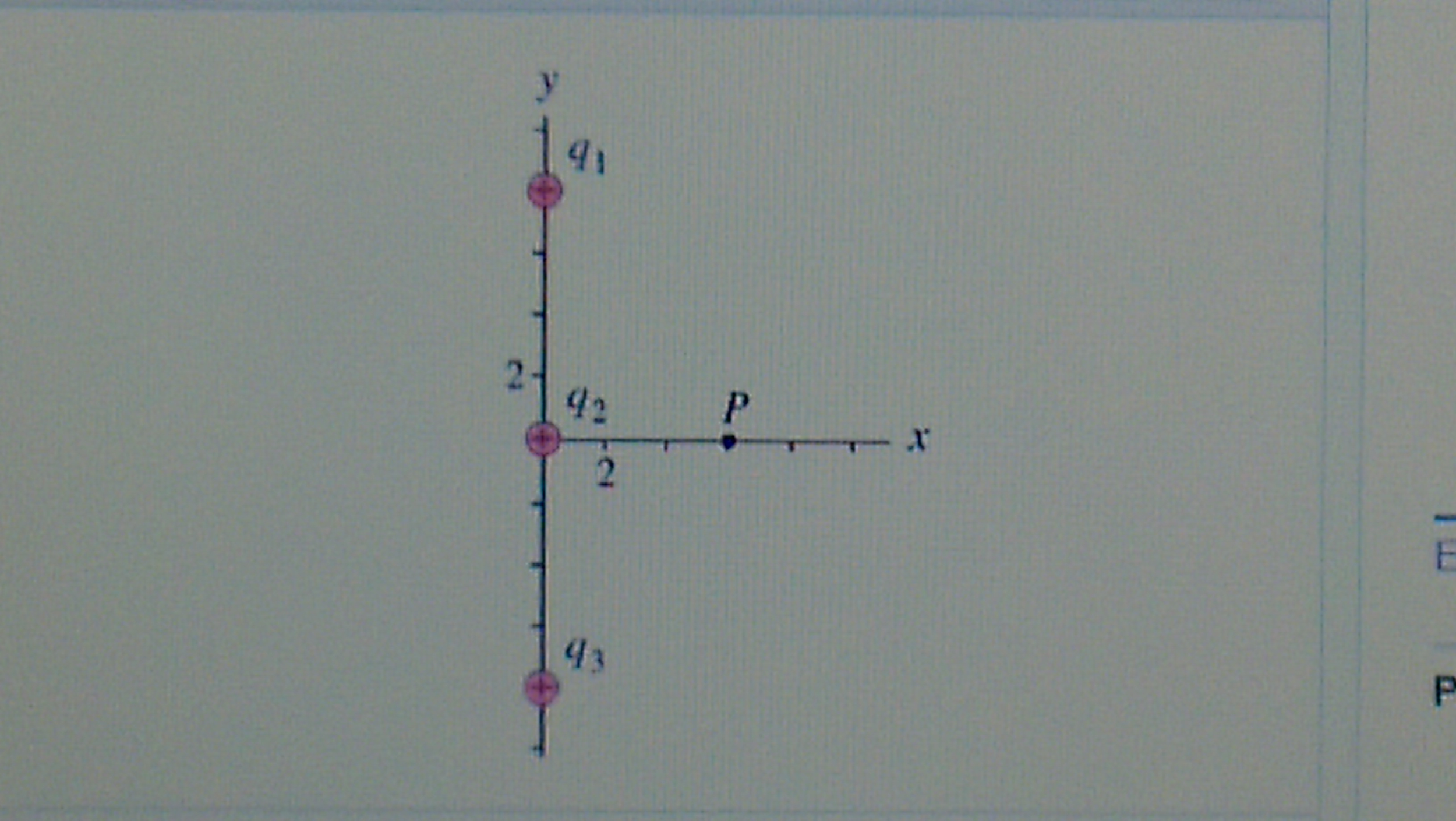# Problem: Three positive point charges (Figure 1) are spaced equally along the y axis (), = 5.00 at , = 5.00 at , and = 5.00at . Find the x and y components of the total electric field and at point, which is located on the x-axis at x = 6.00

###### FREE Expert Solution

In this problem, we'll use the equation of the electric field at a point due to charge, q:

$\overline{){\mathbf{E}}{\mathbf{=}}\frac{\mathbf{k}\mathbf{q}}{{\mathbf{r}}^{\mathbf{2}}}}$, where r is the distance between the charge and the point. k is Coulomb constant.

Let's review what we have:

q1 = 5.00 nC at y = 8 cm

q2 = 5.00 nC at y = 0 cm

q3 = 5.00 nC at y = - 8 cm

All the charges are positive.

This means that the electric field from each charge would be directed away from the charges.

91% (131 ratings)###### Problem Details

Three positive point charges (Figure 1) are spaced equally along the y axis (), = 5.00 at , = 5.00 at , and = 5.00at . Find the x and y components of the total electric field and at point, which is located on the x-axis at = 6.00Frequently Asked Questions

What scientific concept do you need to know in order to solve this problem?

Our tutors have indicated that to solve this problem you will need to apply the Electric Field concept. You can view video lessons to learn Electric Field. Or if you need more Electric Field practice, you can also practice Electric Field practice problems.